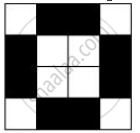# Take Any One Diagonal as a Line of Symmetry and Shade a Few More Squares to Make the Figure Symmetric About a Diagonal. is There More than One Way to Do That? Will the Figure Be Symmetric About Both the Diagonals? - Mathematics

Copy the figure given hereTake any one diagonal as a line of symmetry and shade a few more squares to make the figure symmetric about a diagonal. Is there more than one way to do that? Will the figure be symmetric about both the diagonals?

#### Solution

We can shade a few more squares so as to make the given figure symmetric about any of its diagonals.

Yes, the figure is symmetric about both the diagonals. There is more than one way so as to make the figure symmetric about a diagonal as we can choose any of its 2 diagonals.Concept: Lines of Symmetry for Regular Polygons
Is there an error in this question or solution?

#### APPEARS IN

NCERT Class 7 Maths
Chapter 14 Symmetry
Exercise 14.1 | Q 5 | Page 270